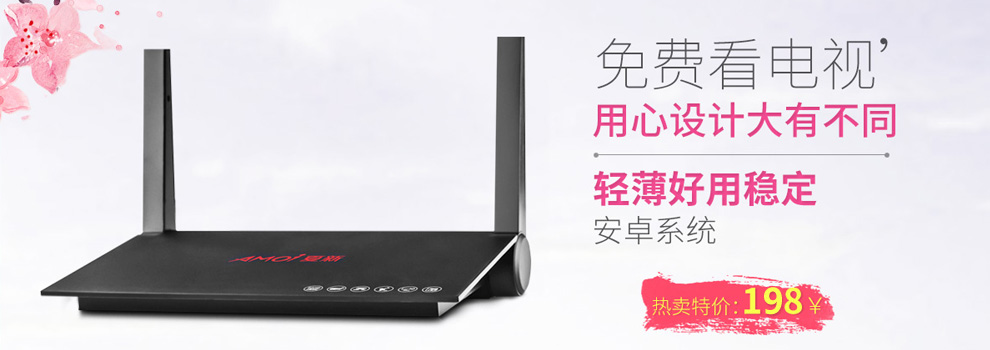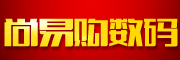20去购物车结算

尚易购智能数码• RMB:
• RMB:
• RMB:
• RMB:
• RMB:
• RMB:
• RMB:
• RMB:

• RMB:
• RMB:
• RMB:
• RMB:
• RMB:
• RMB:
• RMB:
• RMB:
• RMB:
• RMB:
• RMB:
• RMB:
• RMB:
• RMB:
• RMB:
• RMB:
• RMB:
• RMB:
• RMB:
• RMB:
• RMB:
• RMB:
• RMB:
• RMB:
• RMB:
• RMB:
• RMB:
• RMB:

• RMB:
• RMB:
• RMB:
• RMB:1. 品类齐全，轻松购物
2. 多仓直发，极速配送
3. 正品行货，精致服务
4. 天天低价，畅选无忧